## Work and Energy Review

#### Navigate to:

Review Session Home - Topic Listing

Work and Energy - Home || Printable Version || Questions with Links

Answers to Questions:  All || #1-10 || #11-25 || #26-36 || #37-45

 [ #1 | #2 | #3 | #4 | #5 | #6 | #7 | #8 | #9 | #10 | #11 | #12 | #13 | #14 | #15 | #16 | #17 | #18 | #19 | #20 | #21 | #22 | #23 | #24 | #25 | #26 | #27 | #28 | #29 | #30 | #31 | #32 | #33 | #34 | #35 | #36 | #37 | #38 | #39 | #40 | #41 | #42 | #43 | #44 | #45 ]

### Part A: Forced Choice Questions

1. Which of the following statements are true about work? Include all that apply.

1. Work is a form of energy.
2. A Watt is the standard metric unit of work.
3. Units of work would be equivalent to a Newton times a meter.
4. A kg•m2/s2 would be a unit of work.
5. Work is a time-based quantity; it is dependent upon how fast a force displaces an object.
6. Superman applies a force on a truck to prevent it from moving down a hill. This is an example of work being done.
7. An upward force is applied to a bucket as it is carried 20 m across the yard. This is an example of work being done.
8. A force is applied by a chain to a roller coaster car to carry it up the hill of the first drop of the Shockwave ride. This is an example of work being done.
9. The force of friction acts upon a softball player as she makes a headfirst dive into third base. This is an example of work being done.
10. An eraser is tied to a string; a person holds the string and applies a tension force as the eraser is moved in a circle at constant speed. This is an example of work being done.
11. A force acts upon an object to push the object along a surface at constant speed. By itself, this force must NOT be doing any work upon the object.
12. A force acts upon an object at a 90-degree angle to the direction that it is moving. This force is doing negative work upon the object.
13. An individual force does NOT do positive work upon an object if the object is moving at constant speed.
14. An object is moving to the right. A force acts leftward upon it. This force is doing negative work.
15. A non-conservative force is doing work on an object; it is the only force doing work. Therefore, the object will either gain or lose mechanical energy.

 Answer: ACDHIKNO a. TRUE - Work is a form of energy, and in fact it has units of energy. b. FALSE - Watt is the standard metric unit of power; Joule is the standard metric unit of energy. c. TRUE - A N•m is equal to a Joule. d. TRUE - A kg•m2/s2 is a mass unit times a speed squared unit, making it a kinetic energy unit and equivalent to a Joule. e. FALSE - Work is not dependent on how rapidly the force displaces an object; power is time-based and calculated by force multiplied by speed. f. FALSE - Since Superman does not cause a displacement, no work is done; he is merely holding the car to prevent its descent down the hill. g. FALSE - The upward force does not cause the horizontal displacement so this is a NON-example of work. h. TRUE - There is a component of force in the direction of displacement and so this is an example of work. i. TRUE - There is a force and a displacement; the force acts in the opposite direction as the displacement and so this force does negative work. j. FALSE - For uniform circular motion, the force acts perpendicular to the direction of the motion and so the force never does any work upon the object. k. FALSE - This is clearly work - a force is causing an object to be displaced. l. FALSE - If a force acts at a 90-degree angle to the direction of motion, then the force does not do any work at all. Negative work is done when there is a component of force opposite the direction of motion. m. FALSE - There are many instances in which an individual force does positive work and yet the object maintains a constant speed. Consider a force applied to lift an object at constant speed. The force does positive work. Consider a car moving at constant speed along a level surface. The force of the road on the tires does positive work while air resistance does and equal amount of negative work. n. TRUE - A force which acts in a direction opposite the motion of an object will do negative work. o. TRUE - When non-conservative forces do work upon an object, the object will either gain or lose mechanical energy. Mechanical energy is conserved (neither gained nor lost) only when conservative forces do work upon objects.

 Useful Web Links Definition and Mathematics of Work [ #1 | #2 | #3 | #4 | #5 | #6 | #7 | #8 | #9 | #10 | #11 | #12 | #13 | #14 | #15 | #16 | #17 | #18 | #19 | #20 | #21 | #22 | #23 | #24 | #25 | #26 | #27 | #28 | #29 | #30 | #31 | #32 | #33 | #34 | #35 | #36 | #37 | #38 | #39 | #40 | #41 | #42 | #43 | #44 | #45 ]

2. Which of the following statements are true about power? Include all that apply.

1. Power is a time-based quantity.
2. Power refers to how fast work is done upon an object.
3. Powerful people or powerful machines are simply people or machines which always do a lot of work.
4. A force is exerted on an object to move it at a constant speed. The power delivered by this force is the magnitude of the force multiplied by the speed of the object.
5. The standard metric unit of power is the Watt.
6. If person A and person B do the same job but person B does it faster, then person A does more work but person B has more power.
7. The Newton•meter is a unit of power.
8. A 60-kg boy runs up a 2.0 meter staircase in 1.5 seconds. His power is approximately 80 Watt.
9. A 300-Newton force is applied to a skier to drag her up a ski hill at a constant speed of 1.5 m/s. The power delivered by the toe rope is 450 Watts.

 Answer: ABDEI a. TRUE - Power is a rate quantity and thus time-based. b. TRUE - This is the definition of power. c. FALSE - This is not always the case. A machine can do a lot of work but if it fails to do it rapidly, then it is not necessarily powerful. In fact two machines can do the same task (and therefore the same work), yet they can have drastically different power ratings. d. TRUE - An equation for computing work in constant speed situations is P=F•v. e. TRUE - Watt is the unit of power? Yes!! f. FALSE - Vice versa. If two people do the same job, then they're doing the same amount of work. The person who does it fastest generates more power. g. FALSE - A N•m is a Joule and that is a unit of work (not power). Think force (N) times distance (m); that's work (J). h. FALSE - The work would be (m•g)•d or approximately 1200 J. The power is work divided by time - 1200 J/1.5 s = 800 W. i. TRUE - Since force and speed are given, use Power = F•v. The calculation yields 450 W.

 Useful Web Links Power [ #1 | #2 | #3 | #4 | #5 | #6 | #7 | #8 | #9 | #10 | #11 | #12 | #13 | #14 | #15 | #16 | #17 | #18 | #19 | #20 | #21 | #22 | #23 | #24 | #25 | #26 | #27 | #28 | #29 | #30 | #31 | #32 | #33 | #34 | #35 | #36 | #37 | #38 | #39 | #40 | #41 | #42 | #43 | #44 | #45 ]

3. Consider the following physical situations. For each case, determine the angle between the indicated force (in boldface type) and the displacement ("theta" in the work equation).

 a. 0 degrees b. 180 degrees c. 90 degrees d. 30 degrees e. 60 degrees
1. A rightward applied force is used to displace a television set to the right. - Answer: A - 0 degrees
2. The force of friction acts upon a rightward-moving car to bring it to a stop. - Answer: B - 180 degrees
3. A waiter uses an applied force to balance the weight of a tray of plates as he carries the tray across the room. - Answer: C - 90 degrees
4. The force of air resistance acts upon a vertically-falling skydiver. - Answer: B - 180 degrees
5. The force of friction acts upon a baseball player as he slides into third base. - Answer: B - 180 degrees
6. An applied force is used by a freshman to lift a World Civilization book to the top shelf of his locker. - Answer: A - 0 degrees
7. A bucket of water is tied to a string and tension supplies the centripetal force to keep it moving in a circle at constant speed. - Answer: C - 90 degrees
8. An applied force acting at 30-degrees to the horizontal is used to displace an object to the right. - Answer: D - 30 degrees
9. A group of football players use an applied force to push a sled across the grass. - Answer: A - 0 degrees
10. The tension in the elevator cable causes the elevator to rise at a constant speed. - Answer: A - 0 degrees
11. In a physics lab, an applied force is exerted parallel to a plane inclined at 30-degrees in order to displace a cart up the incline. - Answer: A - 0 degrees
12. An applied force is exerted upwards and rightwards at an angle of 30-degrees to the vertical in order to displace an object to the right. - Answer: E - 60 degrees
13. A child rests on the seat of a swing which is supported by the tension in its cables; he swings from the highest position to its lowest position. - Answer: C - 90 degrees

 Answer: See questions above; explanations given below. a. The forward motion is do to the forward pushing; if the force and motion are in the same direction, then the angle is 0 degrees. b. Friction opposes motion and as such does negative work; the angle is 180 degrees. c. The force is vertical and the displacement if horizontal; they make a 90 degree angle. d. Air resistance opposes motion and as such does negative work; the angle is 180 degrees. e. Friction opposes motion and as such does negative work; the angle is 180 degrees. f. The frosh applies an upward force to cause an upward displacement; the angle is 0 degrees. g. For uniform circular motion, the force is inwards and the displacement at each instant is tangent to the circle; these two vectors make a 90 degree angle. h. This is a straightforward question; no tricks here. i. The forward motion is do to the forward pushing; if the force and motion are in the same direction, then the angle is 0 degrees. j. The cable pulls up on the elevator and the elevator is displaced upward; if the force and motion are in the same direction, then the angle is 0 degrees. k. The 30-degree angle is the incline angle, not necessarily the angle between F and d. The force is parallel to the incline and the cart is displaced along the direction of the incline; so the two vectors are in the same direction and the angle between them is 0 degrees. l. Compare the wording of this to part h. This one is tricky because the angle between F and d is 60-degrees. If you missed it, reread the question, paying careful attention to the "with the vertical" part. m. As the child swings, she traces out a circular arc and as such the tension (centripetal) is perpendicular to the direction of motion (tangent).

 Useful Web Links Definition and Mathematics of Work [ #1 | #2 | #3 | #4 | #5 | #6 | #7 | #8 | #9 | #10 | #11 | #12 | #13 | #14 | #15 | #16 | #17 | #18 | #19 | #20 | #21 | #22 | #23 | #24 | #25 | #26 | #27 | #28 | #29 | #30 | #31 | #32 | #33 | #34 | #35 | #36 | #37 | #38 | #39 | #40 | #41 | #42 | #43 | #44 | #45 ]

4. Consider the following physical situations. Identify whether the indicated force (in boldface type) does positive work, negative work or no work.

 a. Positive Work b. Negative Work c. No Work

### Description of Physical Situation

+, -, or no Work

a. A cable is attached to a bucket and the force of tension is used to pull the bucket out of a well.

A. Positive Work

b. Rusty Nales uses a hammer to exert an applied force upon a stubborn nail to drive it into the wall.

A. Positive Work

c. Near the end of the Shockwave ride, a braking system exerts an applied force upon the coaster car to bring it to a stop.

B. Negative Work

d. The force of friction acts upon a baseball player as he slides into third base.

B. Negative Work

e. A busy spider hangs motionless from a silk thread, supported by the tension in the thread.

C. No Work

f. In baseball, the catcher exerts an abrupt applied force upon the ball to stop it in the catcher's mitt.

B. Negative Work

g. In a physics lab, an applied force is exerted parallel to a plane inclined at 30-degrees in order to displace a cart up the incline.

A. Positive Work

h. A pendulum bob swings from its highest position to its lowest position under the influence of the force of gravity.

A. Positive Work

 Answer: See table above; explanations provided below. a. The force is upwards and the displacement is upwards. When the force and the displacement act in the same direction, positive work is done. b. The force is horizontal and the displacement is horizontal. When the force and the displacement act in the same direction, positive work is done. (It is true that the wall is doing negative work upon the nail but this statement is about the hammer's force on the nail.) c. The force is backwards and the displacement is forwards. When the force and the displacement act in the opposite direction, negative work is done. d. The force is backwards and the displacement is forwards. When the force and the displacement act in the opposite direction, negative work is done. e. If the force does not cause the object to be displaced (the object hangs motionless), then no work is done. f. The force is backwards and the displacement is forwards. When the force and the displacement act in the opposite direction, negative work is done. g. The force is upwards and parallel to the incline and the displacement is in the same direction parallel to the incline. When the force and the displacement act in the same direction, positive work is done. h. As the bob swings downwards from its highest position, the motion is downwards (and rightwards); the force is also downwards and as such there is a component of force in the direction of motion. When the force and the displacement act in the same direction, positive work is done. (Note that if the bob was swinging upwards from its lowest position to its highest position, then gravity would be doing negative work.)

 Useful Web Links Definition and Mathematics of Work [ #1 | #2 | #3 | #4 | #5 | #6 | #7 | #8 | #9 | #10 | #11 | #12 | #13 | #14 | #15 | #16 | #17 | #18 | #19 | #20 | #21 | #22 | #23 | #24 | #25 | #26 | #27 | #28 | #29 | #30 | #31 | #32 | #33 | #34 | #35 | #36 | #37 | #38 | #39 | #40 | #41 | #42 | #43 | #44 | #45]

5. Which of the following statements are true about conservative and non-conservative forces? Include all that apply.

1. A force is regarded as a conservative force if it does work but does not remove mechanical energy from a system of objects.
2. A force is regarded as a non-conservative force if it does not add mechanical energy to a system of objects.
3. The force of gravity and elastic (spring) force are both examples of a conservative forces.
4. Applied forces, air resistance, friction forces, and tension are common examples of non-conservative forces.
5. Physicists envy biologists' ability to instill order on the world of animal species through their taxonomic system. So physicists have made a habit of identifying forces as conservative and non-conservative forces in order to instill order on the world of forces.
6. If a non-conservative force acts upon an object, then the object will either gain or lose mechanical energy.
7. If the only forces which do work upon an object are conservative forces, then the object will conserve its mechanical energy.
8. If the sum of an object's KE and PE is remaining constant, then non-conservative forces are NOT doing work.
9. If work is NOT done on an object by a non-conservative force, then the object will experience a transformation of energy from kinetic to potential energy (or vice versa).
10. An object starts from an elevated position with 50 J of potential energy and begins its fall towards the ground. If non-conservative forces can be assumed to NOT do work, then at some point during the fall the object will have 20 J of potential energy and 30 J of kinetic energy.

 Answer: A(sort of) CDGH I(sort of) J a. TRUE (sort of) - If a force does work, yet does not remove mechanical energy from an object, then it is definitely a conservative force. The sort of indicates that a force is also considered a conservative force if it does work and does not add mechanical energy to an object. b. FALSE - If a force does not add mechanical energy to a system of objects, then it is likely a conservative force (provided it doesn't remove mechanical energy either). Non-conservative forces are those which either add or remove energy from a system of objects. c. TRUE - You must know this! d. TRUE - These are all non-conservative forces. You can add normal force to the list as well. e. FALSE - Whether there is envy in a physicist's heart is not for us to tell; the evil found within one's heart is often vast and mysterious ... . We can however definitively say that a physicist classifies forces in order to analyze physical situations in accord with the classification. If only conservative-classified forces do work, then KEi + PEi = KEf + PEf. On the other hand if one or more non-conservative-classified forces are doing work, then KEi + PEi + Wnc = KEf + PEf. f. FALSE - Not only must the force act upon the object, it must also be doing work upon the object. As you sit in your chair, there is a non-conservative force (normal force) acting upon your body. But since it does not do work (it's being assumed that you are not sitting in one of those fancy lounge chairs that has more controls than a TV set), your mechanical energy is not changing. g. TRUE - This is a big principle. You must know this one! h. TRUE - Non conservative forces would alter the total mechanical energy; that is, the PE + KE would not be a constant value. i. TRUE (sort of) - This statement is true (sort of); when only conservative forces are doing work, an object has its kinetic energy transformed into potential energy (or vice versa) without the total amount of the two being altered. It would however be possible that work is not done by a non-conservative force and there be no transformation of energy at all; i.e., the object remains at rest. A conservative force must be doing work in order for there to be a transformation of energy. j. TRUE - One would notice that the PE would begin to drop from 50 J to 0 J and that the KE would increase from 0 J to 50 J. And of course there would be a point at which the PE/KE would be distributed with 20 J to PE and 30 J to KE.

 Useful Web Links Internal (i.e., Conservative) vs. External (i.e., Non-conservative) Forces [ #1 | #2 | #3 | #4 | #5 | #6 | #7 | #8 | #9 | #10 | #11 | #12 | #13 | #14 | #15 | #16 | #17 | #18 | #19 | #20 | #21 | #22 | #23 | #24 | #25 | #26 | #27 | #28 | #29 | #30 | #31 | #32 | #33 | #34 | #35 | #36 | #37 | #38 | #39 | #40 | #41 | #42 | #43 | #44 | #45 ]

6. Which of the following statements are true about kinetic energy? Include all that apply.

1. Kinetic energy is the form of mechanical energy which depends upon the position of an object.
2. If an object is at rest, then it does not have any kinetic energy.
3. If an object is on the ground, then it does not have any kinetic energy.
4. The kinetic energy of an object is dependent upon the weight and the speed of an object.
5. Faster moving objects always have a greater kinetic energy.
6. More massive objects always have a greater kinetic energy.
7. Kinetic energy is a scalar quantity.
8. An object has a kinetic energy of 40 J. If its mass were twice as much, then its kinetic energy would be 80 J.
9. An object has a kinetic energy of 40 J. If its speed were twice as much, then its kinetic energy would be 80 J.
10. Object A has a mass of 1 kg and a speed of 2 m/s. Object B has a mass of 2 kg and a speed of 1 m/s. Objects A and B have the same kinetic energy.
11. An object can never have a negative kinetic energy.
12. A falling object always gains kinetic energy as it falls.
13. A 1-kg object is accelerated from rest to a speed of 2.0 m/s. This object gains 4.0 Joules of kinetic energy.
14. If work is done on an object by a non-conservative force, then the object will either gain or lose kinetic energy.

 Answer: BGHK a. FALSE - Kinetic energy depends upon the speed of the object; potential energy depends upon the position of the object. b. TRUE - Kinetic energy depends upon speed. If there is no speed (the object is at rest), then there is no kinetic energy. c. FALSE - If an object is on the ground, then it does not have potential energy (relative to the ground). d. FALSE (sort of) - Kinetic energy depends upon mass and speed. Two objects of the same mass could have different weights if in a different gravitational field; so it is not appropriate to say that kinetic energy depends upon weight. e. FALSE - Faster moving objects would have more kinetic energy than other objects of the same mass. However, another object could have less speed and make up for this lack of speed in terms of a greater mass. f. FALSE - More massive objects would have more kinetic energy than other objects with the same speed. However, another object could have less mass and make up for this lack of mass in terms of a greater speed. g. TRUE - Kinetic energy does not have a direction associated with it; it is a scalar quantity. h. TRUE - Kinetic energy is directly related to the mass of an object. i. FALSE - Kinetic energy is directly related to the square of the speed of an object. So a doubling of the speed would result in a quadrupling of the kinetic energy - the new KE would be 160 J. j. FALSE - When it comes to kinetic energy, speed is doubly important (recall v2). So in this case, object A would have more kinetic energy. Doing the calculation yields 2 J for object A and 1 J for object B. k. TRUE - Kinetic energy is determined by the equation 0.5•m•v2. the quantity m is always positive. And even if v is negative, v2 will always be positive. Therefore, kinetic energy can never be a negative value. l. FALSE - If an object is falling at a constant velocity (i.e., the air resistance force equals the downward force of gravity), then there is not an increase in kinetic energy. It is true however that free-falling objects always increase their kinetic energy as they fall. m. FALSE - The kinetic energy increases from 0 J to 2 J (0.5•1•22); that's an increase by 2 J. n. FALSE - Such an object will definitely gain or lose mechanical energy but not necessarily kinetic energy.

 Useful Web Links Kinetic Energy [ #1 | #2 | #3 | #4 | #5 | #6 | #7 | #8 | #9 | #10 | #11 | #12 | #13 | #14 | #15 | #16 | #17 | #18 | #19 | #20 | #21 | #22 | #23 | #24 | #25 | #26 | #27 | #28 | #29 | #30 | #31 | #32 | #33 | #34 | #35 | #36 | #37 | #38 | #39 | #40 | #41 | #42 | #43 | #44 | #45 ]

7. Which of the following statements are true about potential energy? Include all that apply.

1. Moving objects cannot have potential energy.
2. Potential energy is the energy stored in an object due to its position.
3. Both gravitational and elastic potential energy are dependent upon the mass of an object.
4. The gravitational potential energy of an object is dependent upon the mass of the object.
5. If the mass of an elevated object is doubled, then its gravitational potential energy will be doubled as well.
6. Gravitational potential energy is lost as objects free-fall to the ground.
7. The higher that an object is, the more potential energy which it will have.
8. The unit of measurement for potential energy is the Joule.
9. A 1-kg mass at a height of 1 meter has a potential energy of 1 Joule.
10. A 1-kg object falls from a height of 10 m to a height of 6 m. The final potential energy of the object is approximately 40 J.
11. If work is done on an object by a non-conservative force, then the object will either gain or lose potential energy.

 Answer: BDEFGH a. FALSE - Potential energy has nothing to do with speed; an object could be moving at an elevated position. It is this elevation above zero level which gives an object potential energy. b. TRUE - This is the definition of potential energy. c. FALSE - Gravitational potential energy is dependent upon the mass of the object (PEgrav = m•g•h) but elastic potential energy is dependent upon the spring constant and the compression or stretch length of the spring (PEelastic = 0.5•k•x2). d. TRUE - The equation states that PEgrav = m•g•h; PE is dependent upon mass. e. TRUE - The equation states that PEgrav = m•g•h; if the h is doubled, then the PE will be doubled as well. f. TRUE - As objects free-fall, the height (h) decreases; subsequently, the PE decreases. g. TRUE - The equation states that PEgrav = m•g•h; PE is directly related to height. h. TRUE - The Joule (abbrev. J) is the standard metric unit of energy - all forms of energy. i. FALSE - The final potential energy is calculated as PE = m•g•h = (1 kg)•(~10 m/s/s)•(1 m) = ~10 J. j. FALSE - The final potential energy is calculated as PE = m•g•h = (1 kg)•(~10 m/s/s)•(6 m) = ~60 J; the loss in potential energy during this 4-m fall is -40 J. k. FALSE - The object will either gain or lose mechanical energy, but not necessarily potential energy.

 Useful Web Links Potential Energy [ #1 | #2 | #3 | #4 | #5 | #6 | #7 | #8 | #9 | #10 | #11 | #12 | #13 | #14 | #15 | #16 | #17 | #18 | #19 | #20 | #21 | #22 | #23 | #24 | #25 | #26 | #27 | #28 | #29 | #30 | #31 | #32 | #33 | #34 | #35 | #36 | #37 | #38 | #39 | #40 | #41 | #42 | #43 | #44 | #45 ]

8. Which of the following statements are true about mechanical energy? Include all that apply.

1. The total amount of mechanical energy of an object is the sum of its potential energy and the kinetic energy.
2. Heat is a form of mechanical energy.
3. The mechanical energy of an object is always conserved.
4. When non-conservative forces do work, energy is transformed from kinetic to potential (or vice versa), but the total mechanical energy is conserved.
5. A bowling ball is mounted from a ceiling by way of a strong cable. It is drawn back and released, allowed to swing as a pendulum. As it swings from its highest position to its lowest position, the total mechanical energy is mostly conserved.
6. When a friction force does work on an object , the total mechanical energy of that object is changed.
7. The total mechanical energy of an object remains constant if the only forces doing work on the object are conservative forces.
8. If an object gains mechanical energy, then one can be certain that a non-conservative force is doing work.

 Answer: AEFGH a. TRUE - This is the definition of mechanical energy. b. FALSE - Heat or thermal energy is a non-mechanical form of energy. Potential and kinetic energy are the only forms of mechanical energy. c. FALSE - The mechanical energy of an object is only conserved if non-conservative forces do not do work upon the object. d. FALSE- If a non-conservative force does work upon an object, then the total mechanical energy of that object is changed. Energy will not be conserved. e. TRUE - Tension does not do work upon the object and so the total mechanical energy is conserved. The presence of air resistance (a non-conservative force) does a little work and so one might notice a very slight change in mechanical energy. f. TRUE - Friction is a non-conservative force and thus alters the total mechanical energy of an object. g. TRUE - This is the conservation of energy principle and one that you need to firmly understand. h. TRUE - If there is any change in the total mechanical energy of an object (whether a gain or a loss), then you know for certain that there is a non-conservative force doing work.

 Useful Web Links Mechanical Energy [ #1 | #2 | #3 | #4 | #5 | #6 | #7 | #8 | #9 | #10 | #11 | #12 | #13 | #14 | #15 | #16 | #17 | #18 | #19 | #20 | #21 | #22 | #23 | #24 | #25 | #26 | #27 | #28 | #29 | #30 | #31 | #32 | #33 | #34 | #35 | #36 | #37 | #38 | #39 | #40 | #41 | #42 | #43 | #44 | #45 ]

9. Rank these four objects in increasing order of kinetic energy, beginning with the smallest.

 Object A Object B Object C Object D m = 5.0 kg v = 4.0 m/s h = 2.0 m m = 10.0 kg v = 2.0 m/s h = 3.00 m m = 1.0 kg v = 5.0 m/s h = 5.0 m m = 5.0 kg v = 2.0 m/s h = 4.0 m

 Answer: D < C < B < A This is probably best done by performing a calculation of KE and comparing the results: Object A: KE = 0.5•(5.0 kg)•(4.0 m/s)2 = 40. J Object B: KE = 0.5•(10.0 kg)•(2.0 m/s)2 = 20. J Object C: KE = 0.5•(1.0 kg)•(5.0 m/s)2 = ~13 J (12.5 J) Object D: KE = 0.5•(5.0 kg)•(2.0 m/s)2 = 10. J The order is evident once the calculations are performed.

 Useful Web Links Kinetic Energy [ #1 | #2 | #3 | #4 | #5 | #6 | #7 | #8 | #9 | #10 | #11 | #12 | #13 | #14 | #15 | #16 | #17 | #18 | #19 | #20 | #21 | #22 | #23 | #24 | #25 | #26 | #27 | #28 | #29 | #30 | #31 | #32 | #33 | #34 | #35 | #36 | #37 | #38 | #39 | #40 | #41 | #42 | #43 | #44 | #45 ]

10. Rank these four objects in increasing order of potential energy, beginning with the smallest.

 Object A Object B Object C Object D m = 5.0 kg v = 4.0 m/s h = 2.0 m m = 10.0 kg v = 2.0 m/s h = 3.00 m m = 1.0 kg v = 5.0 m/s h = 5.0 m m = 5.0 kg v = 2.0 m/s h = 4.0 m

 Answer: C < A < D < B This is probably best done by performing a calculation of PE and comparing the results.  Using the approximation that g = ~10 m/s/s gives much quicker results. Object A: PE = (5.0 kg)•(~10 m/s2)•(2.0 m) = ~100 J Object B: PE = (10.0 kg)•(~10 m/s2)•(3.00 m) = ~300 J Object C: PE = (1.0 kg)•(~10 m/s2)•(5.0 m) = ~50 J Object D: PE = (5.0 kg)•(~10 m/s2)•(4.0 m) = ~200 J The order is evident once the calculations are performed.

 Useful Web Links Potential Energy [ #1 | #2 | #3 | #4 | #5 | #6 | #7 | #8 | #9 | #10 | #11 | #12 | #13 | #14 | #15 | #16 | #17 | #18 | #19 | #20 | #21 | #22 | #23 | #24 | #25 | #26 | #27 | #28 | #29 | #30 | #31 | #32 | #33 | #34 | #35 | #36 | #37 | #38 | #39 | #40 | #41 | #42 | #43 | #44 | #45 ]

NOTE: The next 15 questions presume that the value of g is ~10 m/s/s.

11. A 1200 kg car and a 2400 kg car are lifted to the same height at a constant speed in a auto service station. Lifting the more massive car requires ____ work.

 a. less b. the same c. twice as much d. four times as much e. more than 4 times as much

 Answer: C The amount of work done by a force to displace an object is found from the equation W = F*d*cos(Theta) The force required to raise the car at constant speed is equivalent to the weight (m*g) of the car. Since the 2400-kg car weighs 2X as much as the 1200-kg car, it would require twice as much work to lift it the same distance.

 Useful Web Links Definition and Mathematics of Work | Calculating the Amount of Work Done by Forces [ #1 | #2 | #3 | #4 | #5 | #6 | #7 | #8 | #9 | #10 | #11 | #12 | #13 | #14 | #15 | #16 | #17 | #18 | #19 | #20 | #21 | #22 | #23 | #24 | #25 | #26 | #27 | #28 | #29 | #30 | #31 | #32 | #33 | #34 | #35 | #36 | #37 | #38 | #39 | #40 | #41 | #42 | #43 | #44 | #45 ]

12. An arrow is drawn back so that 50 Joules of potential energy is stored in the stretched bow and string. When released, the arrow will have a kinetic energy of ____ Joules.

 a. 50 b. more than 50 c. less than 50

 Answer: A A drawn arrow has 50 J of stored energy due to the stretch of the bow and string. When released, this energy is converted into kinetic energy such that the arrow will have 50 J of kinetic energy upon being fired. Of course, this assumes no energy is lost to air resistance, friction or any other non-conservative forces and that the arrow is shot horizontally.

 Useful Web Links Analysis of Situations in Which Mechanical Energy is Conserved [ #1 | #2 | #3 | #4 | #5 | #6 | #7 | #8 | #9 | #10 | #11 | #12 | #13 | #14 | #15 | #16 | #17 | #18 | #19 | #20 | #21 | #22 | #23 | #24 | #25 | #26 | #27 | #28 | #29 | #30 | #31 | #32 | #33 | #34 | #35 | #36 | #37 | #38 | #39 | #40 | #41 | #42 | #43 | #44 | #45 ]

13. A child lifts a box up from the floor. The child then carries the box with constant speed to the other side of the room and puts the box down. How much work does he do on the box while walking across the floor at constant speed?

 a. zero J b. more than zero J c. more information needed to determine

 Answer: A For any given situation, the work done by a force can be calculated using the equation W = F*d*cos(Theta) where F is the force doing the work, d is the displacement of the object, and Theta is the angle between the force and the displacement. In this specific situation, the child is applying an upward force on the box (he is carrying it) and the displacement of the box is horizontal. The angle between the force (vertical) and the displacement (upward) vectors is 90 degrees. Since the cosine of 90-degrees is 0, the child does not do any work upon the box. A detailed discussion of a similar situation (the waiter and the tray of food) can be found at The Physics Classroom.

 Useful Web Links Definition and Mathematics of Work [ #1 | #2 | #3 | #4 | #5 | #6 | #7 | #8 | #9 | #10 | #11 | #12 | #13 | #14 | #15 | #16 | #17 | #18 | #19 | #20 | #21 | #22 | #23 | #24 | #25 | #26 | #27 | #28 | #29 | #30 | #31 | #32 | #33 | #34 | #35 | #36 | #37 | #38 | #39 | #40 | #41 | #42 | #43 | #44 | #45]

14. A 1000-kg car is moving at 40.0 km/hr when the driver slams on the brakes and skids to a stop (with locked brakes) over a distance of 20.0 meters. How far will the car skid with locked brakes if it is traveling at 120. km/hr?

 a. 20.0 m b. 60.0 m c. 90.0 m d. 120. m e. 180. m

 Answer: E When a car skids to a stop, the work done by friction upon the car is equal to the change in kinetic energy of the car. Work is directly proportional to the displacement of the car (skidding distance) and the kinetic energy is directly related to the square of the speed (KE=0.5*m*v2). For this reason, the skidding distance is directly proportional to the square of the speed. So if the speed is tripled from 40 km/hr to 120 km/hr, then the stopping distance is increased by a factor of 9 (from 20 m to 9*20 m; or 180 m). A detailed discussion of the distance-speed squared relationship can be found at The Physics Classroom.

 Useful Web Links Analysis of Situations Involving External Forces [ #1 | #2 | #3 | #4 | #5 | #6 | #7 | #8 | #9 | #10 | #11 | #12 | #13 | #14 | #15 | #16 | #17 | #18 | #19 | #20 | #21 | #22 | #23 | #24 | #25 | #26 | #27 | #28 | #29 | #30 | #31 | #32 | #33 | #34 | #35 | #36 | #37 | #38 | #39 | #40 | #41 | #42 | #43 | #44 | #45 ]

15. A platform diver weighs 500 N. She steps off a diving board that is elevated to a height of 10 meters above the water. The diver will possess ___ Joules of kinetic energy when she hits the water.

 a. 10 b. 500 c. 510 d. 5000 e. more than 5000 .

 Answer: D The use of the work-energy theorem and a simple analysis will yield the solution to this problem. Initially, there is only PE; finally, there is only KE. Assuming negligible air resistance, the kinetic energy of the diver upon hitting the water is equal to the potential energy of the diver on top of the board. PEi = KEf m*g*hi = KEf Substituting 500 N for m*g (500 N is the weight of the diver, not the mass) and 10 m for h will yield the answer of 5000 J.

 Useful Web Links Potential Energy | Kinetic Energy | Analysis of Situations in Which Mechanical Energy is Conserved [ #1 | #2 | #3 | #4 | #5 | #6 | #7 | #8 | #9 | #10 | #11 | #12 | #13 | #14 | #15 | #16 | #17 | #18 | #19 | #20 | #21 | #22 | #23 | #24 | #25 | #26 | #27 | #28 | #29 | #30 | #31 | #32 | #33 | #34 | #35 | #36 | #37 | #38 | #39 | #40 | #41 | #42 | #43 | #44 | #45]

16. A ball is projected into the air with 100 J of kinetic energy. The kinetic energy is transformed into gravitational potential energy on the path towards the peak of its trajectory. When the ball returns to its original height, its kinetic energy is ____ Joules. Do consider the effects of air resistance

 a. less than 100 b. 100 c. more than 100 d. not enough information given

 Answer: A During any given motion, if non-conservative forces do work upon the object, then the total mechanical energy will be changed. If non-conservative forces do negative work (i.e., Fnc*d*cos(Theta) is a negative number), then the final TME is less than the initial TME. In this case, air resistance does negative work to remove energy from the system. Thus, when the ball returns to its original height, their is less TME than immediately after it was thrown. At this same starting height, the PE is the same as before. The reduction in TME is made up for by the fact that the kinetic energy has been reduced; the final KE is less than the initial KE.

 Useful Web Links Analysis of Situations Involving External Forces [ #1 | #2 | #3 | #4 | #5 | #6 | #7 | #8 | #9 | #10 | #11 | #12 | #13 | #14 | #15 | #16 | #17 | #18 | #19 | #20 | #21 | #22 | #23 | #24 | #25 | #26 | #27 | #28 | #29 | #30 | #31 | #32 | #33 | #34 | #35 | #36 | #37 | #38 | #39 | #40 | #41 | #42 | #43 | #44 | #45 ]

17. During a construction project, a 2500 N object is lifted high above the ground. It is released and falls 10.0 meters and drives a post 0.100 m into the ground. The average impact force on the object is ____ Newtons.

 a. 2500 b. 25000 c. 250,000 d. 2,500,000

 Answer: C The use of the work-energy theorem and a simple analysis will yield the solution to this problem. Initially, there is only PE; finally, there is neither PE nor KE; non-conservative work has been done by an applied force upon the falling object. The work-energy equation can be written as follows. PEi + Wnc = 0 PEi = - Wnc m*g*hi = - F*d*cos(Theta) Substituting 2500 N for m*g (2500 N is the weight of the driver, not the mass); 10.0 m for h; 0.100 m for the displacement of the falling object as caused by the upward applied force exerted by the post; and 90 degrees for Theta (the angle between the applied force and the displacement of the falling object) will yield the answer of 250000 N for F.

 Useful Web Links Analysis of Situations Involving External Forces [ #1 | #2 | #3 | #4 | #5 | #6 | #7 | #8 | #9 | #10 | #11 | #12 | #13 | #14 | #15 | #16 | #17 | #18 | #19 | #20 | #21 | #22 | #23 | #24 | #25 | #26 | #27 | #28 | #29 | #30 | #31 | #32 | #33 | #34 | #35 | #36 | #37 | #38 | #39 | #40 | #41 | #42 | #43 | #44 | #45 ]

18. A 10-Newton object moves to the left at 1 m/s. Its kinetic energy is approximately ____ Joules.

 a. 0.5 b. 1 c. 10 d. more than 10

 Answer: A The KE of any object can be computed if the mass (m) and speed (v) are known. Simply use the equation KE=0.5*m*v2 In this case, the 10-N object has a mass of approximately 1 kg (use Fgrav = m*g). The speed is 1 m/s. Now plug and chug to yield KE of approximately 0.5 J.

 Useful Web Links Kinetic Energy [ #1 | #2 | #3 | #4 | #5 | #6 | #7 | #8 | #9 | #10 | #11 | #12 | #13 | #14 | #15 | #16 | #17 | #18 | #19 | #20 | #21 | #22 | #23 | #24 | #25 | #26 | #27 | #28 | #29 | #30 | #31 | #32 | #33 | #34 | #35 | #36 | #37 | #38 | #39 | #40 | #41 | #42 | #43 | #44 | #45 ]

19. Luke Autbeloe stands on the edge of a roof throws a ball downward. It strikes the ground with 100 J of kinetic energy. Luke now throws another identical ball upward with the same initial speed, and this too falls to the ground. Neglecting air resistance, the second ball hits the ground with a kinetic energy of ____ J.

 a. less than 100 b. 100 c. 200 d. more than 200 e. none of these

 Answer: B Quite surprisingly to many, each ball would hit the ground with the same speed. In each case, the PE+KE of the balls immediately after being thrown is the same (they are thrown with the same speed from the same height). Upon hitting the ground, they must also have the same PE+KE. Since the PE is zero (on the ground) for each ball, it stands to reason that their KE is also the same. That's a little physics and a lot of logic - and try not to avoid the logic part by trying to memorize the answer.

 Useful Web Links Potential Energy | Kinetic Energy | Analysis of Situations in Which Mechanical Energy is Conserved [ #1 | #2 | #3 | #4 | #5 | #6 | #7 | #8 | #9 | #10 | #11 | #12 | #13 | #14 | #15 | #16 | #17 | #18 | #19 | #20 | #21 | #22 | #23 | #24 | #25 | #26 | #27 | #28 | #29 | #30 | #31 | #32 | #33 | #34 | #35 | #36 | #37 | #38 | #39 | #40 | #41 | #42 | #43 | #44 | #45 ]

20. An object at rest may have __________.

 a. speed b. velocity c. acceleration d. energy e. all of these

 Answer: D An object at rest absolutely cannot have speed or velocity or acceleration. However, an object at rest could have energy if there is energy stored due to its position; for example, there could be gravitational or elastic potential energy.

 Useful Web Links Potential Energy | Kinetic Energy | Mechanical Energ [ #1 | #2 | #3 | #4 | #5 | #6 | #7 | #8 | #9 | #10 | #11 | #12 | #13 | #14 | #15 | #16 | #17 | #18 | #19 | #20 | #21 | #22 | #23 | #24 | #25 | #26 | #27 | #28 | #29 | #30 | #31 | #32 | #33 | #34 | #35 | #36 | #37 | #38 | #39 | #40 | #41 | #42 | #43 | #44 | #45 ]

21. A 50-kg platform diver hits the water below with a kinetic energy of 5000 Joules. The height (relative to the water) from which the diver dove was approximately ____ meters.

 a. 5 b. 10 c. 50 d. 100

 Answer: B The kinetic energy of the diver upon striking the water must be equal to the original potential energy. Thus, m*g*hi = KEf (50 kg)*(~10 m/s/s)*h = 5000 J So, h = ~10 m

 Useful Web Links Potential Energy | Kinetic Energy | Analysis of Situations in Which Mechanical Energy is Conserved [ #1 | #2 | #3 | #4 | #5 | #6 | #7 | #8 | #9 | #10 | #11 | #12 | #13 | #14 | #15 | #16 | #17 | #18 | #19 | #20 | #21 | #22 | #23 | #24 | #25 | #26 | #27 | #28 | #29 | #30 | #31 | #32 | #33 | #34 | #35 | #36 | #37 | #38 | #39 | #40 | #41 | #42 | #43 | #44 | #45 ]

22. A job is done slowly, and an identical job is done quickly. Both jobs require the same amount of ____, but different amounts of ____. Pick the two words which fill in the blanks in their respective order.

 a. energy, work b. power, work c. work, energy d. work, power e. power, energy f. force, work g. power, force h. none of these

 Answer: D Power refers to the rate at which work is done. Thus, doing two jobs - one slowly and one quickly - involves doing the same job (i.e., the same work and same force) at different rates or with different power.

 Useful Web Links Definition and Mathematics of Work | Power [ #1 | #2 | #3 | #4 | #5 | #6 | #7 | #8 | #9 | #10 | #11 | #12 | #13 | #14 | #15 | #16 | #17 | #18 | #19 | #20 | #21 | #22 | #23 | #24 | #25 | #26 | #27 | #28 | #29 | #30 | #31 | #32 | #33 | #34 | #35 | #36 | #37 | #38 | #39 | #40 | #41 | #42 | #43 | #44 | #45 ]

23. Which requires more work: lifting a 50.0 kg crate a vertical distance of 2.0 meters or lifting a 25.0 kg crate a vertical distance of 4.0 meters?

 a. lifting the 50 kg crate b. lifting the 25 kg crate c. both require the same amount of work

Work involves a force acting upon an object to cause a displacement. The amount of work done is found by multiplying F*d*cos(Theta). The equation can be used for these two motions to find the work.

 Lifting a 50 kg crate vertically 2 meters W = (~500 N)*(2 m)*cos(0) W = ~1000 N (Note: The weight of a 50-kg object is approximately 500 N; it takes 500 N to lift the object up.) Lifting a 25 kg crate vertically 4 meters W = (~250 N)*(4 m)*cos(0) W = ~1000 N (Note: The weight of a 25-kg object is approximately 250 N; it takes 250 N to lift the object up.)

 Useful Web Links Definition and Mathematics of Work [ #1 | #2 | #3 | #4 | #5 | #6 | #7 | #8 | #9 | #10 | #11 | #12 | #13 | #14 | #15 | #16 | #17 | #18 | #19 | #20 | #21 | #22 | #23 | #24 | #25 | #26 | #27 | #28 | #29 | #30 | #31 | #32 | #33 | #34 | #35 | #36 | #37 | #38 | #39 | #40 | #41 | #42 | #43 | #44 | #45 ]

24. A 50.0 kg crate is lifted to a height of 2.0 meters in the same time as a 25.0 kg crate is lifted to a height of 4 meters. The rate at which energy is used (i.e., power) in raising the 50.0 kg crate is ____ as the rate at which energy is used to lift the 25.0 kg crate.

 a. twice as much b. half as much c. the same

 Answer: C The power is the rate at which work is done (or energy is used). Power is found by dividing work by time. It requires the same amount of work to do these two jobs (see question #23) and the same amount of time. Thus, the power is the same for both tasks.

 Useful Web Links Definition and Mathematics of Work | Potential Energy
[ #1 | #2 | #3 | #4 | #5 | #6 | #7 | #8 | #9 | #10 | #11 | #12 | #13 | #14 | #15 | #16 | #17 | #18 | #19 | #20 | #21 | #22 | #23 | #24 | #25 | #26 | #27 | #28 | #29 | #30 | #31 | #32 | #33 | #34 | #35 | #36 | #37 | #38 | #39 | #40 | #41 | #42 | #43 | #44 | #45 ]

25. Using 1000. J of work, a small object is lifted from the ground floor to the third floor of a tall building in 20.0 seconds. What power was required in this task?

 a. 20 W b. 50 W c. 100 W d. 1000 W e. 20000 W

 Answer: B This is a relatively simple plug-and-chug into the equation P=W/t with W=1000. J and t=20.0 s.

[ #1 | #2 | #3 | #4 | #5 | #6 | #7 | #8 | #9 | #10 | #11 | #12 | #13 | #14 | #15 | #16 | #17 | #18 | #19 | #20 | #21 | #22 | #23 | #24 | #25 | #26 | #27 | #28 | #29 | #30 | #31 | #32 | #33 | #34 | #35 | #36 | #37 | #38 | #39 | #40 | #41 | #42 | #43 | #44 | #45 ]

### Part B: Straightforward Computational Problems

26. Approximate the work required lift a 2.5-kg object to a height of 6.0 meters. PSYW

 Answer: ~150 J The work done upon an object is found with the equation W = F*d*cos(Theta) In this case, the d=6.0 m; the F=24.5 N (it takes 24.5 N of force to lift a 2.5-kg object; that's the weight of the object), and the angle between F and d (Theta) is 0 degrees. Substituting these values into the above equation yields W = F*d*cos(Theta) = (24.5 N)*(6 m)*cos(0) = ~150 J (147 J)

 Useful Web Links Definition and Mathematics of Work [ #1 | #2 | #3 | #4 | #5 | #6 | #7 | #8 | #9 | #10 | #11 | #12 | #13 | #14 | #15 | #16 | #17 | #18 | #19 | #20 | #21 | #22 | #23 | #24 | #25 | #26 | #27 | #28 | #29 | #30 | #31 | #32 | #33 | #34 | #35 | #36 | #37 | #38 | #39 | #40 | #41 | #42 | #43 | #44 | #45]

27. A student applies a force to a cart to pull it up an inclined plane at a constant speed during a physics lab. A force of 20.8 N is applied parallel to the incline to lift a 3.00-kg loaded cart to a height of 0.450 m along an incline which is 0.636-m long. Determine the work done upon the cart and the subsequent potential energy change of the cart. PSYW

 Answer: 13.2 J There are two methods of solving this problem. The first method involves using the equation W = F*d*cos(Theta) where F=20.8 N, d=0.636 m, and Theta=0 degrees. (The angle theta represents the angle betwee the force and the displacement vector;  since the force is applied parallel to the incline, the angle is zero.) Substituting and solving yields W = F*d*cos(Theta) = (20.8 N)*(0.636 m)*cos(0) = 13.2 J. The second method is to recognize that the work done in pulling the cart along the incline at constant speed changes the potential energy of the cart. The work done equals the potential energy change. Thus, W=Delta PE = m*g*(delta h) = (3.00 kg)*(9.8 m/s/s)*(0.45 m) = 13.2 J

 Useful Web Links Definition and Mathematics of Work | Potential Energy [ #1 | #2 | #3 | #4 | #5 | #6 | #7 | #8 | #9 | #10 | #11 | #12 | #13 | #14 | #15 | #16 | #17 | #18 | #19 | #20 | #21 | #22 | #23 | #24 | #25 | #26 | #27 | #28 | #29 | #30 | #31 | #32 | #33 | #34 | #35 | #36 | #37 | #38 | #39 | #40 | #41 | #42 | #43 | #44 | #45 ]

28. Eddy, whose mass is 65.0-kg, climbs up the 1.60-meter high stairs in 1.20 s. Approximate Eddy's power rating. PSYW

 Answer: P = 849 Watts Eddy's power is found by dividing the work which he does by the time in which he does it. The work done in elevating his 65.0-kg mass up the stairs is determined using the equation W = F*d*cos(Theta) where F = m*g = 637 N (the weight of the 65.0 kg object), d =1.60 m and Theta = 0 degrees (the angle between the upward force and the upward displacement). Solving for W yields 1019.2 Joules. Now divide the work by the time to determine the power: P = W/t = (1019.2 J)/(1.20 s) = 849 Watts

 Useful Web Links Definition and Mathematics of Work | Power [ #1 | #2 | #3 | #4 | #5 | #6 | #7 | #8 | #9 | #10 | #11 | #12 | #13 | #14 | #15 | #16 | #17 | #18 | #19 | #20 | #21 | #22 | #23 | #24 | #25 | #26 | #27 | #28 | #29 | #30 | #31 | #32 | #33 | #34 | #35 | #36 | #37 | #38 | #39 | #40 | #41 | #42 | #43 | #44 | #45 ]

29. A 51.7-kg hiker ascends a 43.2-meter high hill at a constant speed of 1.20 m/s. If it takes 384 s to climb the hill, then determine ... . PSYW

1. kinetic energy change of the hiker.
2. the potential energy change of the hiker.
3. the work done upon the hiker.
4. the power delivered by the hiker.

 Answers: Delta KE = 0 J Delta PE = +21900 J W = +21900 J P = 57.0 Watts a. The speed of the hiker is constant so there is no change in kinetic energy - 0 J. b. The potential energy change can be found by subtracting the initial PE (0 J) from the final PE (m*g*hf). The final potential energy is 21888 J [from (51.7 kg)*(9.8 m/s/s)*(43.2 m)] and the initial potential energy is 0 J. So Delta PE = +21900 J (rounded from 21888 J). c. The work done upon the hiker can be found using the work-energy theorem. The equation reduces to Wnc = PEf (PEi = 0 J since the hiker starts on the ground; and KEi = KEf since the speed is constant; these two terms can be dropped from the equation since they are equal). The final potential energy is 21888 J [from (51.7 kg)*(9.8 m/s/s)*(43.2 m)]. So W = +21900 J (rounded from 21888 J). d. The power of the hiker can be found by dividing the work by the time. P=W/t=(21888 J)/(384 s) = 57.0 Watts

 Useful Web Links Potential Energy | Kinetic Energy | Definition and Mathematics of Work | Power [ #1 | #2 | #3 | #4 | #5 | #6 | #7 | #8 | #9 | #10 | #11 | #12 | #13 | #14 | #15 | #16 | #17 | #18 | #19 | #20 | #21 | #22 | #23 | #24 | #25 | #26 | #27 | #28 | #29 | #30 | #31 | #32 | #33 | #34 | #35 | #36 | #37 | #38 | #39 | #40 | #41 | #42 | #43 | #44 | #45]

30. An 878-kg car skids to a stop across a horizontal surface over a distance of 45.2 m. The average force acting upon the car is 7160 N. Determine ... . PSYW

1. the work done upon the car.
2. the initial kinetic energy of the car.
3. the acceleration of the car.
4. the initial velocity of the car.

 Answers: W = -324000 J KEi = +324000 J a = -8.16 m/s/s vi = 27.2 m/s a. The work done upon the car can be found using the equation W = F*d*cos(Theta) where F=7160 N, d=45.2 m, and Theta=180 degrees (the force is in the opposite direction as the displacement). Substituting and solving yields -323632 J (rounded to -324000 J). b. The initial kinetic energy can be found using the work-energy theorem. The equation reduces to KEi + Wnc = 0 (PEi and PEf = 0 J since the car is on the ground; and KEf = 0 J since the car is finally stopped). Rearrange the equation and it takes the form KEi = -Wnc . So KEi = +324000 J (rounded from +323632 J). c. The acceleration of the car can be found using Newton's second law of motion: Fnet = m*a The friction force is the net force (since the up and down forces balance) and the mass is 878 kg. Substituting and solving yields a = -8.16 m/s/s. d. The initial velocity of the car can be found using the KE equation: KE = 0.5*m*v2 where m=878 kg and KEi=323632 J. Substituting and solving for velocity (v) yields v = 27.2 m/s. (A kinematic equation could be also used to find the initial velocity.)

 Useful Web Links Calculating Work | Analysis of Situations Involving External Forces [ #1 | #2 | #3 | #4 | #5 | #6 | #7 | #8 | #9 | #10 | #11 | #12 | #13 | #14 | #15 | #16 | #17 | #18 | #19 | #20 | #21 | #22 | #23 | #24 | #25 | #26 | #27 | #28 | #29 | #30 | #31 | #32 | #33 | #34 | #35 | #36 | #37 | #38 | #39 | #40 | #41 | #42 | #43 | #44 | #45 ]

31. A 510-kg roller coaster car starts at a height of 32.0 m. Assuming negligible energy losses to friction and air resistance, determine the PE, KE, and speed of the car at the various locations (A, B, C, D, and E) along the track.

 Location Height (m) PE* (J) KE* (J) velocity (m/s) Start 32.0 160 000 J 0 J 0 A 28.0 140 000 J 20 000 J 8.9 m/s B 11.0 55 000 J 105 000 J 20.5 m/s C 20.0 100 000 J 60 000 J 15.5 m/s D 5.0 25 000 J 135 000 J 23.2 m/s E 15.0 75 000 J 85 000 J 18.4 m/s F 0 0 J 160 000 J 25.3 m/s

*rounded to the second significant digit
 Answers: See above table (answers in red) The potential energy for every row can be found using the equation PE = m*g*h where m=510 kg and g = 9.8 m/s/s. In the first row, the total mechanical energy (KE + PE) equals 160 000 J (rounded). Since no work is done by non-conservative forces, the total mechanical energy must be 160 000 J in all the other rows. So the KE can be computed by subtracting the PE from 160 000 J. The velocity can be found using the equation: KE = 0.5*m*v2 where m=510 kg.  The algebraic rearrangement of this equation results in v = SQRT(2*KE/m).

 Useful Web Links Potential Energy | Kinetic Energy | Analysis of Situations in Which Mechanical Energy is Conserved [ #1 | #2 | #3 | #4 | #5 | #6 | #7 | #8 | #9 | #10 | #11 | #12 | #13 | #14 | #15 | #16 | #17 | #18 | #19 | #20 | #21 | #22 | #23 | #24 | #25 | #26 | #27 | #28 | #29 | #30 | #31 | #32 | #33 | #34 | #35 | #36 | #37 | #38 | #39 | #40 | #41 | #42 | #43 | #44 | #45 ]

32. A 65.8-kg skier accelerates down an icy hill from an original height of 521 meters. Use the work-energy theorem to determine the speed at the bottom of the hill if...

a. ... no energy is lost or gained due to friction, air resistance and other non-conservative forces. PSYW

b. ... 1.40*105 J of energy are lost due to external forces. PSYW

 Answers: (a) v = 101 m/s; (b) v = 77.2 m/s a. Use the work energy theorem: KEi + PEi + Wnc = KEf + PEf The PEf can be dropped from the equation since the skier finishes on the ground at zero height. The KEi can also be dropped since the skier starts from rest. The Wnc term is dropped since it is said that no work is done by non-conservative (external) forces. The equation simplifies to PEi = KEf The expressions for KE (0.5*m*v2) and PE (m*g*h) can be substituted into the equation: m*g*h = 0.5*m*vf2 where m=65.8 kg, h=521 m, g=9.8 m/s/s. Substituting and solving for vf yields 101 m/s. b. This equation can be solved in a similar manner, except that now the Wext term is -140000 J. So the equation becomes m*g*h - 140000 J = 0.5*m*vf2 Now substituting and solving for vf yields 77.2 m/s.

 Useful Web Links Analysis of Situations in Which Mechanical Energy is Conserved | Analysis of Situations Involving External Forces [ #1 | #2 | #3 | #4 | #5 | #6 | #7 | #8 | #9 | #10 | #11 | #12 | #13 | #14 | #15 | #16 | #17 | #18 | #19 | #20 | #21 | #22 | #23 | #24 | #25 | #26 | #27 | #28 | #29 | #30 | #31 | #32 | #33 | #34 | #35 | #36 | #37 | #38 | #39 | #40 | #41 | #42 | #43 | #44 | #45]

33. Use the work-energy theorem to determine the force required to stop a 988-kg car moving at a speed of 21.2 m/s if there is a distance of 45.7 m in which to stop it. PSYW

 Answer: F = 4.86*103 N The work energy theorem can be written as KEi + PEi + Wnc = KEf + PEf The PEi and PEf can be dropped from the equation since they are both 0 (the height of the car is 0 m). The KEf can also be dropped for the same reason (the car is finally stopped). The equation simplifies to KEi + Wnc = 0 The expressions for KE (0.5*m*v2) and Wnc (F*d*cos[Theta]) can be substituted into the equation: 0.5*m*vi2 + F*d*cos[Theta] = 0 where m=988 kg, vi=21.2 m/s, d=45.7 m, and Theta = 180 degrees. Substituting and solving for F yields 4.86*103 N.

 Useful Web Links Kinetic Energy | Analysis of Situations Involving External Forces [ #1 | #2 | #3 | #4 | #5 | #6 | #7 | #8 | #9 | #10 | #11 | #12 | #13 | #14 | #15 | #16 | #17 | #18 | #19 | #20 | #21 | #22 | #23 | #24 | #25 | #26 | #27 | #28 | #29 | #30 | #31 | #32 | #33 | #34 | #35 | #36 | #37 | #38 | #39 | #40 | #41 | #42 | #43 | #44 | #45 ]

### Part C: Work-Energy Bar Charts, Analysis, and Conceptual Reasoning

34. Consider the following physical situations. Identify the forces which do work upon the indicated object (in boldface type) and categorize them as conservative or non-conservative forces. Then indicate whether the total mechanical energy of the object changes; it it changes, then indicate whether the change is a positive or negative change. Finally, indicate whether the potential energy and the kinetic energy changes; if PE or KE changes, then indicate whether the change is a positive or negative change.

### Description of Physical Situation

Identity of Forces

Which Do Work:

Change in TME
Change in PE
Change in KE
Conserv-
ative
Non-
Conserv-
ative
Yes

(+ or -)

No
Yes

(+ or -)

No
Yes

(+ or -)

No

a. A force is applied to move a physics cart from the floor to the top of an inclined plane at a constant speed.

Gravity
Applied
+

+

No

b. A physics student scurries up a flight of stairs at constant speed.

Gravity
Applied or Normal
+

+

No

c. In a moment of unsupervised phun, a physics student hoists herself onto a staircase banister and accelerates down the banister. Ignore all friction forces.

Gravity
None

No
-

+

d. A ball is dropped from rest from on the top of a hill and falls to the ground below. Ignore air resistance.

Gravity
None

No
-

+

e. A ball leaves top of a hill with a large horizontal velocity. It falls to the ground below. Ignore air resistance.

Gravity
None

No
-

+

f. A Hot Wheels car is at rest at an elevated position along an inclined plane; it is released and rolls to a position along the ground. Ignore air resistance.

Gravity
None

No
-

+

g. A Hot Wheels car is in motion at the bottom of a hill when it hits a computer diskette box and skids to a stop.

None
Applied
-

No
-

h. A pendulum bob swings from its highest position to its lowest position.

Gravity
None

No
-

+

i. A physics cart is released from rest at an elevated position along an inclined plane; it is released and rolls to a position along the incline approximately 5 cm from the bottom.

Gravity
None

No
-

+

 Answer: See table above Note that whenever a non-conservative force is doing work (as in a, b, g), the total mechanical energy is changing. However, when the only forces which do work are conservative forces, the total mechanical energy will remaining constant (i.e., be conserved). In such cases, energy will change form from potential to kinetic energy (or vice versa); yet the total amount of the two forms would not be changed. Potential energy can be considered to change if an object changes its height; if the height decreases, then the potential energy decreases; it the height increases, then the potential energy increases. Kinetic energy can be considered to change if an object changes its speed; if the speed decreases, then the kinetic energy decreases; it the speed increases, then the kinetic energy increases.

 Useful Web Links Analysis of Situations in Which Mechanical Energy is Conserved | Analysis of Situations Involving External Forces [ #1 | #2 | #3 | #4 | #5 | #6 | #7 | #8 | #9 | #10 | #11 | #12 | #13 | #14 | #15 | #16 | #17 | #18 | #19 | #20 | #21 | #22 | #23 | #24 | #25 | #26 | #27 | #28 | #29 | #30 | #31 | #32 | #33 | #34 | #35 | #36 | #37 | #38 | #39 | #40 | #41 | #42 | #43 | #44 | #45 ]

35. The diagrams below depict a physical situation. Analyze each situation and construct work-energy bar charts.

 Description of Physical Situation Work-Energy Bar Chart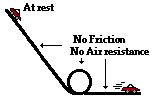a. A Hot Wheels car starts from rest on top of an inclined plane and rolls down the incline through a loop and along a horizontal surface. Ignore the effect of friction and air resistance on the car.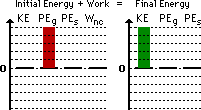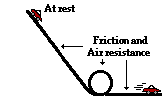b. A Hot Wheels car starts from rest on top of an inclined plane and rolls down the incline through a loop and along a horizontal surface. Friction and air resistance have a significant effect on the car.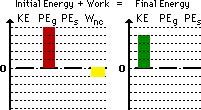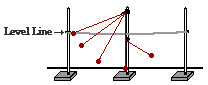c. A pendulum bob is mounted on top of a lab pole and drawn back to a string which it tied between two other poles. The pendulum bob is released from rest. Upon reaching the vertical, the string hits a barrier and a new pivot point is established as the bob continues in motion along an upward trajectory. Ignore the effect of air resistance.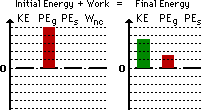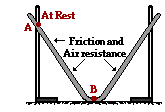d. A track is constructed by stretching a grooved and pliable material between two lab poles. A metal ball starts from rest at point A and rolls along the groove to point B. Friction and air resistance have an effect upon the ball's motion.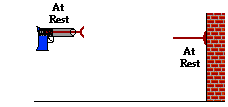e. A dart is placed in a dart gun and the springs are compressed. The trigger is pulled and the dart is projected towards a wall. The dart collides with the wall and sticks to it. Ignore the effect of friction and air resistance.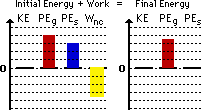f. Ben Laborin applies a force to push a crate from the bottom of an inclined plane to the top at a constant speed.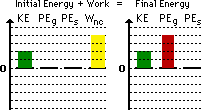Answer: See above table; explanations given below. There are a couple of major principles behind these bar charts: First, the initial energy (KEi + PEgi + PEsi) plus the work done by non-conservative forces (Wnc) must equal the final energy (KEf + PEgf + PEsf); thus the heights of the bars on the left side of the chart must equal the height of the bars on the left side of the chart. Second, the bars must appropriately depict the form of energy and the relative amount for both the initial and final state. The above charts are not the only means of appropriately representing the motion. Other renditions which adhere to these two basic principles would be considered correct. a. The car is initially at rest (no KE) and has some height above the floor (there is PEg). The car is moving at the bottom of the track (there is KE) but it does not have any height (no PEg). Since there is no friction nor air resistance, the Wnc term is zero. b. Part b is similar to part a with the exception that friction and air resistance are to be considered in part b. So the analysis involves a consideration of the Wnc term. The Wnc term is negative since friction and air resistance are forces which oppose the motion of an object. The result is that energy has been removed from the system and the final kinetic energy bar is less than the initial potential energy bar. c. If the tabletop (or floor) is considered the zero level, then the bob has gravitational potential energy in both its initial and final states. Yet the final PEg is less than the initial PEg since the final height (as shown) is less than the initial height. Since the air resistance is negligible, the Wnc term is zero. The difference in potential energy must be made up for in the form of kinetic energy. The bob would still be moving at the final position as shown. d. This analysis is identical to part a except that the car has been replaced by a ball. e. The dart is initially above the ground (the assumed zero-level) and finishes above the ground but a little lower than the initial height; thus, there is some PEg to begin with and a little less PEg in the final state. The springs are initially compressed, so there is some initial PEs. The wall does work upon the dart to stop it; this would be negative work since the force is exerted in a direction opposite the motion of the dart. Initially the dart is not moving; so there is no initial KE. And after hitting the wall, it is not moving as well. f. The worker is applying a force to displace the crate; this is a non-conservative force and so there will be a Wnc term. Since this applied force is in the direction of motion, the Wnc term will be positive. The crate is moving (it has KE) at a constant speed; since the speed is constant, the amount of KE is the same in the initial state as in the final state. Initially there is no gravitational potential energy (PEg) since the crate starts on the ground; in the final state, there is some gravitational potential energy (PEg) since the crate is at an elevated position.

 Useful Web Links Bar Chart Illustrations [ #1 | #2 | #3 | #4 | #5 | #6 | #7 | #8 | #9 | #10 | #11 | #12 | #13 | #14 | #15 | #16 | #17 | #18 | #19 | #20 | #21 | #22 | #23 | #24 | #25 | #26 | #27 | #28 | #29 | #30 | #31 | #32 | #33 | #34 | #35 | #36 | #37 | #38 | #39 | #40 | #41 | #42 | #43 | #44 | #45 ]

36. Several physical situations are described below. For each situation, simplify the work-energy equation by canceling any zero terms and any energy terms (whether KE or PE) which are unchanging. Explain each term which gets canceled. The first problem is done as an example.

 Description of Physical Situation Simplification of Work-Energy Equation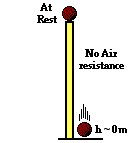a. A ball starts from rest on top of a tall pillar and falls to the ground below. Assume the effect of air resistance is negligible. PEi = KEf (Since initially at rest, KEi = 0 and cancels. Since the final height is 0, PEf = 0 and cancels. Since non-conservative forces are not doing work, Wnc = 0)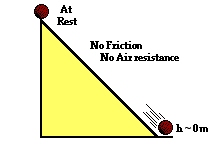b. A ball starts from rest at an elevated position along an inclined plane and rolls to the ground below. Assume that the effect of friction and air resistance is negligible. PEi = KEf (Initially the ball is at rest, KEi = 0 and cancels. Since the final height is 0, PEf = 0 and cancels. Since non-conservative forces are not doing work, Wnc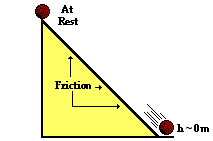c. A ball starts from rest at an elevated position along an inclined plane and rolls to the ground below. Consider the effect of friction and air resistance. PEi + Wnc = KEf (Initially the ball is at rest, KEi = 0 and cancels. Since the final height is 0, PEf = 0 and cancels. Friction is a non-conservative forces and it does work so Wnc does not cancel.)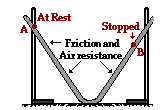d. A track is constructed by stretching a grooved and pliable material between two lab poles. A metal ball starts from rest at point A and rolls to point B. Friction and air resistance have an effect upon the ball's motion. PEi + Wnc = PEf (The ball is at rest in both its initial and final states, so both KEi = 0 and KEf = 0 and cancels. Friction and air resistance are non-conservative forces and do work so Wnc does not cancel.)e. A pendulum bob is mounted on top of a lab pole and drawn back to a string which it tied between two other poles. The pendulum bob is released from rest. Upon reaching the vertical, the string hits a barrier and a new pivot point is established as the bob continues in motion along an upward trajectory. Ignore the effect of air resistance. PEi = KEf + PEf (Initially the ball is at rest, KEi = 0 and cancels. At the point shown in the diagram as the final state, the ball has both height and would still be moving; so neither final energy term would cancel. The only non-conservative force present - tension - does not do work since it is directed at 90-degrees to the direction of motion; so Wnc cancels.)f. A Hot Wheels car starts from rest on top of an inclined plane and rolls down the incline through a loop and along a horizontal surface. Friction and air resistance have a significant effect on the car. PEi + Wnc = KEf (Initially the car is at rest, KEi = 0 and cancels. Since the final height is 0, PEf = 0 and cancels. Friction is a non-conservative forces and it does work so Wnc does not cancel.)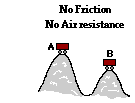g. An unattended hot dog wagon starts from rest and rolls down a hill and up a second hill. Ignore the effect of friction and air resistance. PEi = KEf + PEf (Initially the wagon is at rest, KEi = 0 and cancels. The wagon has height in its initial state, so the PEi term does not cancel. At the point shown in the diagram as the final state, the wagon has both height and would still be moving; so neither final energy term would cancel. There is no friction nor air resistance and the normal force does not do work since it is perpendicular to the displacement; so Wnc cancels.)h. A roller coaster car is already in motion on the top of the first drop and rolls along the track over a couple of hills. Ignore the effect of friction and air resistance. PEi = KEf + PEf (Initially the car is at rest, KEi = 0 and cancels. The car has height in its initial state, so the PEi term does not cancel. At the point shown in the diagram as the final state, the car has both height and would still be moving; so neither final energy term would cancel. There is no friction nor air resistance and the normal force does not do work since it is perpendicular to the displacement; so Wnc cancels.)i. A cross-country skier is in motion on top of a small hill. He skis down the hill into the valley and up a second smaller hill. He uses his poles to propel himself during the entire motion. Ignore the effect of friction and air resistance. KEi + PEi + Wnc = KEf + PEf (Initially, the skier has height and motion and so neither initial energy term will cancel. The skier is using his poles to propel himself so there is a non-conservative force doing work; the Wnc term does not cancel. In the final state, the skier is moving and has height (presuming that the zero level is the valley) and so neither final energy term will cancel.

 Useful Web Links Analysis of Situations in Which Mechanical Energy is Conserved | Analysis of Situations Involving External Forces [ #1 | #2 | #3 | #4 | #5 | #6 | #7 | #8 | #9 | #10 | #11 | #12 | #13 | #14 | #15 | #16 | #17 | #18 | #19 | #20 | #21 | #22 | #23 | #24 | #25 | #26 | #27 | #28 | #29 | #30 | #31 | #32 | #33 | #34 | #35 | #36 | #37 | #38 | #39 | #40 | #41 | #42 | #43 | #44 | #45 ]

### Part D: Complex Analysis and Problem-Solving

37. A 21.3-kg child positions himself on an inner-tube which is suspended by a 7.28-m long rope attached to a strong tree limb. The child and tube is drawn back until it makes a 17.4-degree angle with the vertical. The child is released and allowed to swing to and from. Assuming negligible friction, determine the child's speed at his lowest point in the trajectory. PSYW

 Answer: 2.56 m/s This is an example of energy transformation from potential energy at the highest point (the point of release) to kinetic energy at the lowest position. Since gravity is the only force doing work (tension acts perpendicular to the displacement so it does not do work), the total mechanical energy is conserved. So the energy conservation equation will be used. KEi + PEi = KEf + PEf Since the child starts from rest, the KEi term can be canceled. And if we assign the lowest position as the zero-level, then the PEf term can be canceled from the equation. The equation can be rewritten as PEi = KEf By substituting in expressions for kinetic and potential energy, the equation becomes m • g • hi = 0.5 • m • vf2 The mass cancels from each side of the equation, and algebra can be used to manipulate the equation to solve for vf. vf = SQRT( 2 • g • hi )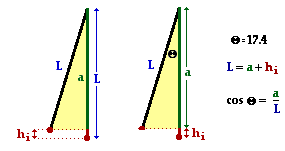The crux of the problem becomes determining the initial height of the child. The length of the cables and the angle to which it is drawn back will be essential information in determining the initial height. The diagram at the right depicts the physical situation. The length of the cable is the hypotenuse of a triangle; the length of the side adjacent to the 17.4-degree angle can be determined using the cosine function. This adjacent side (a) is shorter than the length of the cable by an amount equal to the initial height (hi). The work is shown below: cos(17.4 deg) = a/L a = L • cos(17.4 deg) = 6.95 m hi = L - a = 7.28 m - 6.95 mm = 0.333 m Once hi is found, it can be substituted into the above equation to solve for the final velocity: vf = SQRT ( 2 • 9.8 m/s/s • 0.33 m) = SQRT(6.53 m/s) = 2.56 m/s

 Useful Web Links Analysis of Situations in Which Mechanical Energy is Conserved | Analysis of Situations Involving External Forces [ #1 | #2 | #3 | #4 | #5 | #6 | #7 | #8 | #9 | #10 | #11 | #12 | #13 | #14 | #15 | #16 | #17 | #18 | #19 | #20 | #21 | #22 | #23 | #24 | #25 | #26 | #27 | #28 | #29 | #30 | #31 | #32 | #33 | #34 | #35 | #36 | #37 | #38 | #39 | #40 | #41 | #42 | #43 | #44 | #45 ]

38. A baseball player catches a 163-gram baseball which is moving horizontally at a speed of 39.8 m/s. Determine the force which she must apply to the baseball if her mitt recoils a horizontal distance of 25.1 cm. PSYW

 Answer: 514 N This is an example of work being done by a non-conservative force (the applied force of the mitt) upon a baseball in order to change its kinetic energy. So Wnc = Change in KE The change in kinetic energy can be computed by subtracting the initial value (0.5 • m • vi2) from the final value (0 J) . Change in KE = KEf - KEi = 0 J - 0.5 • (0.163 kg) • (39.8 m/s)2 = -129 J The force can be determined by setting this value equal to the work and using the expression for work into the equation: Wnc = -129 J F • d • cos(theta) = -129 J F • (0.251 m) • cos(180 deg) = -129 J F = 514 N

 Useful Web Links Analysis of Situations in Which Mechanical Energy is Conserved | Analysis of Situations Involving External Forces [ #1 | #2 | #3 | #4 | #5 | #6 | #7 | #8 | #9 | #10 | #11 | #12 | #13 | #14 | #15 | #16 | #17 | #18 | #19 | #20 | #21 | #22 | #23 | #24 | #25 | #26 | #27 | #28 | #29 | #30 | #31 | #32 | #33 | #34 | #35 | #36 | #37 | #38 | #39 | #40 | #41 | #42 | #43 | #44 | #45 ]

39. A 62.9-kg downhill skier is moving with a speed of 12.9 m/s as he starts his descent from a level plateau at 123-m height to the ground below. The slope has an angle of 14.1 degrees and a coefficient of friction of 0.121. The skier coasts the entire descent without using his poles; upon reaching the bottom he continues to coast to a stop; the coefficient of friction along the level surface is 0.623. How far will he coast along the level area at the bottom of the slope? PSYW

During the entire descent down the hill, gravity is doing work on the skier and friction is doing negative work on the skier. Friction is a non-conservative force and will alter the total mechanical energy of the skier. The equation to be used is

KEi + PEi + Wnc = KEf + PEf

If we designate the level area at the bottom of the slope as the zero level of potential energy, then PEf is 0 J. Since the skier eventually stops (due to the effect of friction along the level area), the KEf is 0 J. So the above equation becomes

KEi + PEi + Wnc = 0

The Wnc term has two parts; there is friction doing along the inclined plane and friction doing work along the level surface. Since these two sections of the motion have different normal forces and friction coefficients (and therefore friction forces), they will have to be treated separately. The graphic below depicts the free-body diagrams and the means by which the friction force can be determined.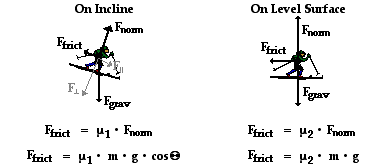By substituting values of mu and mass and g and theta into the above equations, one finds that the friction values are

 On Incline Ffrict = 72.3 N On Level Surface Ffrict = 384 N

These forces act upon the skier over different distances. In the case of the inclined plane, the distance (d) can be computed from the given incline angle and the initial height. The relationship is depicted in the diagram below. The sine function is used to relate the angle to the initial height and the distance along the incline. In the case of the level surface, the distance is the unknown quantity (x) which this problem calls for.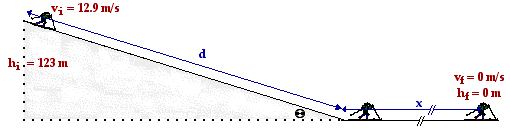The distance d along the incline is

d = hi / sin(theta) = 123 m / sin(14.1 deg) = 505 m

Now substitutions can be made into the work-energy equation and algebraic manipulation can be performed to solve for x:

KEi + PEi + Wnc = 0

KEi + PEi + Wincline + Wlevel = 0

0.5•(62.9 kg)•(12.9 m/s)2 + (62.9 kg)•(9.8 m/s)•(123 m) + (72.3 N)•(505 m)•cos(180 deg) + (384 N)•(x)•cos(180 deg) = 0 J

5233 J + 75820 J - 36512 J - 384 x = 0 J

44541 J = 384 x

116 m = x

 Useful Web Links Analysis of Situations in Which Mechanical Energy is Conserved | Analysis of Situations Involving External Forces [ #1 | #2 | #3 | #4 | #5 | #6 | #7 | #8 | #9 | #10 | #11 | #12 | #13 | #14 | #15 | #16 | #17 | #18 | #19 | #20 | #21 | #22 | #23 | #24 | #25 | #26 | #27 | #28 | #29 | #30 | #31 | #32 | #33 | #34 | #35 | #36 | #37 | #38 | #39 | #40 | #41 | #42 | #43 | #44 | #45 ]

40. A 29.1-kg sledder is traveling along a level area with a speed of 8.96 m/s when she approaches a gentle incline which makes an angle of 12.5 degrees with the horizontal. If the coefficient of friction between the sled and the incline is 0.109, then what will be her speed at the bottom of the inclined plane, located 8.21 m above the top of the incline. PSYW

 Answer: 12.7 m/s This problem is similar to the above. During the entire descent down the hill, gravity is doing work on the sledder and friction is doing negative work on the sledder. Friction is a non-conservative force and will alter the total mechanical energy of the sledder. The equation to be used is KEi + PEi + Wnc = KEf + PEf If we designate the level area at the bottom of the slope as the zero level of potential energy, then PEf is 0 J. So the above equation becomes KEi + PEi + Wnc = KEf 0.5•m•vi2 + m•g•hi + F•d•cos(theta) = 0.5•m•vf2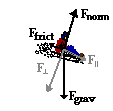The Wnc term results from friction acting upon the sledder. The force of friction is given by the equation Ffrict = mu • Fnorm. For inclined planes the normal force is equal to the perpendicular component of the weight vector (see diagram at the right). This component is equal to the expression m•g•cos(theta) where theta is the incline angle (12.5 degrees). So the force of friction is Ffrict = mu•m•g•cos(theta) Ffrict = (0.109)•(29.1 kg)•(9.8 m/s/s)•cos(12.5 deg) = 30.3 N The work done by the friction force occurs over the entire length of the inclined plane. This distance is related to the initial height and the incline angle by the equation sin(theta) = hi/d (see diagram below). Using 12.5 degrees for theta and 8.21 m for the initial height, the distance along the incline (d) can be determined to be 37.9 m. The diagram below depicts the physical situation.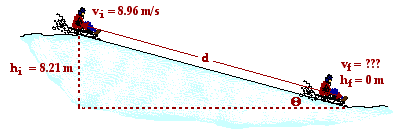Now substitutions can be made into the earlier stated equation and algebra manipulations can be performed to determine the final speed. The work is shown below. 0.5•m•vi2 + m•g•hi + F•d•cos(theta) = 0.5•m•vf2 0.5•(29.1 kg)•(8.96 m/s)2 + (29.1 kg)•(9.8 m/s/s)•(8.21 m) + (30.3 N)(37.9 m)•cos(180 deg)= 0.5•(29.1 kg)•vf2 1168 J + 2341 J - 1148 J = (14.6 kg)•vf2 162.3 m2/s2 = vf2 vf = SQRT(162.3 m2/s2) = 12.7 m/s

 Useful Web Links Analysis of Situations in Which Mechanical Energy is Conserved | Analysis of Situations Involving External Forces [ #1 | #2 | #3 | #4 | #5 | #6 | #7 | #8 | #9 | #10 | #11 | #12 | #13 | #14 | #15 | #16 | #17 | #18 | #19 | #20 | #21 | #22 | #23 | #24 | #25 | #26 | #27 | #28 | #29 | #30 | #31 | #32 | #33 | #34 | #35 | #36 | #37 | #38 | #39 | #40 | #41 | #42 | #43 | #44 | #45 ]

41. A 221-gram ball is thrown at an angle of 17.9 degrees and a speed of 36.7 m/s from the top of a 39.8-m high cliff. Determine the impact speed of the ball when it strikes the ground. Assume negligible air resistance. PSYW

 Answer: 46.1 m/s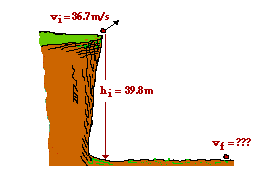Here is a situation in which the only force doing work -gravity - is a conservative force; so the total mechanical energy of the system is conserved. The conservation of energy equation can be used. KEi + PEi = KEf + PEf If we assign the ground below the cliff to be the zero-level of potential energy, then the PEf term can be canceled from the equation. KEi + PEi = KEf Expressions for kinetic and potential energy can be substituted into the above equation to yield. 0.5•m•vi2 + m•g•hi = 0.5•m•vf2 By dividing each term of the equation by m, the mass can be canceled. 0.5 • vi2 + g • hi = 0.5 • vf2 Now known values can be substituted into this equation and the final velocity can be determined. The work is shown here: 0.5•(36.7 m/s)2 + (9.8 m/s/s)•(39.8 m) = 0.5 • vf2 673.4 m2/s2 + 390.0 m2/s2 = 0.5 • vf2 1063 m2/s2 = 0.5 • vf2 2127 m2/s2 = vf2 SQRT(2127 m2/s2) = vf vf = 46.1 m/s

 Useful Web Links Analysis of Situations in Which Mechanical Energy is Conserved | Analysis of Situations Involving External Forces [ #1 | #2 | #3 | #4 | #5 | #6 | #7 | #8 | #9 | #10 | #11 | #12 | #13 | #14 | #15 | #16 | #17 | #18 | #19 | #20 | #21 | #22 | #23 | #24 | #25 | #26 | #27 | #28 | #29 | #30 | #31 | #32 | #33 | #34 | #35 | #36 | #37 | #38 | #39 | #40 | #41 | #42 | #43 | #44 | #45 ]

42. Claire deAisles has just completed her shopping at the grocery food store. She accidentally bumps her 42.5-kg cart, setting it in motion from rest down a hill inclined at 14.9 degrees. Upon descending a distance of 9.27 meters along the inclined plane, the cart hits a tree stump (which was placed in the parking lot for the sole purpose of this problem). A 0.295-kg can of tomato soup is immediately hurled from the moving cart and heads towards Will N. Tasue's brand new Lexus. Upon striking the Lexus, the tomato soup can creates a dent with a depth of 3.16 cm. Noah Formula, who is watching the entire incident and fixing to do some physics, attempts to calculate the average force which the Lexus applies to the soup can. Assume negligible air resistance and friction forces and help Noah out. PSYW

 Answer: 218 N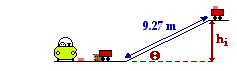Here's an example of a physical situation in which gravity first acts to do work and convert the potential energy of a soup can (and the grocery cart) into kinetic energy; then a non-conservative force - the force of the Lexus applied to the soup can - serves to change the kinetic energy of the soup can. The problem is best analyzed if the cart itself is ignored and the focus becomes the soup can. The soup can begins with potential energy on top of the hill and finishes with no mechanical energy. The situation is depicted at the right. The relevant equation becomes the work-energy equation: KEi + PEi + Wnc = KEf + PEf The final potential and kinetic energy can be canceled and the initial kinetic energy can be canceled (the can starts from rest). PEi + Wnc = 0 J The expressions for potential energy and work can be substituted into the above equation to derive: m•g•hi + F•d•cos(theta) = 0 J The initial height of the soup can is related to the angle of incline and the length along the incline according to the equation sin(theta) = hi/L The values of theta (14.9 degrees) and L (9.27 m) can be substituted into this equation and hi is found to be 2.38 m. This hi value can be substituted into the work-energy equation along with the values of m (0.295 kg) and d (0.0316 m). The force can then be calculated. The work is shown here. (0.295 kg)•(9.8 m/s/s)•(2.38 m) + F•(0.0316 m)•cos(180 deg) = 0 J 6.89 J - (0.0316m ) • F = 0 J 6.89 J = (0.0316m ) • F F = 218 N (Note there is an assumption in this problem that the collision with the Lexus chassis brings the soup can to a final rest position; This is likely not the case since a soup can typically does not become lodged inside the Lexus, but rather rebounds with a post-impact velocity. Yet without this assumption or further information, the problem cannot be solved.)

 Useful Web Links Analysis of Situations in Which Mechanical Energy is Conserved | Analysis of Situations Involving External Forces [ #1 | #2 | #3 | #4 | #5 | #6 | #7 | #8 | #9 | #10 | #11 | #12 | #13 | #14 | #15 | #16 | #17 | #18 | #19 | #20 | #21 | #22 | #23 | #24 | #25 | #26 | #27 | #28 | #29 | #30 | #31 | #32 | #33 | #34 | #35 | #36 | #37 | #38 | #39 | #40 | #41 | #42 | #43 | #44 | #45 ]

43. Pete Zaria applies a 11.9-Newton force to a 1.49-kg mug of root beer in order to accelerate it from rest over a distance of 1.42-m. Once released, how far will the mug slide along the counter top if the coefficient of friction is 0.728? PSYW

 Answer: 0.170 m Here is an instance in which there is only horizontal motion and a non-conservative force is doing work to change the kinetic energy of the mug. The entire motion will have to be divided into two parts - the part in which both friction and applied force act upon the mug (Pete is pushing it from position A to position B) and the part in which the mug is slowing down under the sole influence of friction (from position B to position C). The work-energy theorem (W = Delta KE) will be used to analyze each part.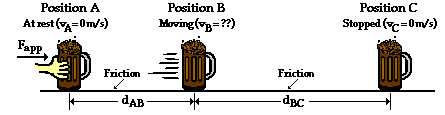From position A to position B, Pete is pushing the mug and the net force upon the mug is simply the applied force minus the friction force (see free-body diagram below). From position B to position C, friction is the unbalanced force and acts as the net force (see free-body diagram below).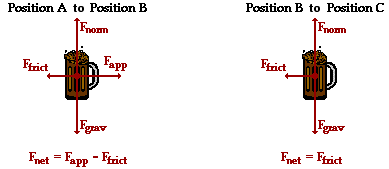The force of friction is related to the normal force (= m•g) and the coefficient of friction (0.728). The Ffrict is Ffrict = mu•Fnorm = (0.728) • (1.49 kg) • (9.8 m/s/s) = 10.6 N This means that the net force from A to B is 11.9 N - 10.6 N = 1.27 N. The net force from B to C is 10.6 N. From A to B, the work done equals the kinetic energy change. So the kinetic energy at position B is KEB = W = F • d • cos(theta) = (1.27 N) • (1.42 m) • cos(0 deg) = 1.80 J From B to C, the mug will lose this same amount of kinetic energy as friction works upon it to bring it to a stop. So the work done from B to C is -1.80 J. W = Change in KE F • d • cos(theta) = -1.80 J (10.6 N) • (d) • cos(180 deg) = -1.80 J - (10.6 N) • (d) = -1.80 J d = 0.170 m

 Useful Web Links Analysis of Situations in Which Mechanical Energy is Conserved | Analysis of Situations Involving External Forces [ #1 | #2 | #3 | #4 | #5 | #6 | #7 | #8 | #9 | #10 | #11 | #12 | #13 | #14 | #15 | #16 | #17 | #18 | #19 | #20 | #21 | #22 | #23 | #24 | #25 | #26 | #27 | #28 | #29 | #30 | #31 | #32 | #33 | #34 | #35 | #36 | #37 | #38 | #39 | #40 | #41 | #42 | #43 | #44 | #45 ]

44. Suzie Lovtaski has a mass of 49.7 kg. She is at rest on top of a hill with a height of 92.6 m and an incline angle of 19.2 degrees. She coasts down the hill to the bottom and eventually comes to a stop; she never uses her poles to apply a force. The coefficient of friction is 0.0873 along the hill and 0.527 along the horizontal surface at the bottom. What total distance will Suzie coast (include both incline and level surface)? PSYW

This problem is very similar to question #39 and can be treated in much the same way. There is a non-conservative force - friction - doing work upon the skier. This force will alter the total mechanical energy of the skier. The equation to be used is

KEi + PEi + Wnc = KEf + PEf

If we designate the level area at the bottom of the slope as the zero level of potential energy, then PEf is 0 J. Since Suzie eventually stops (due to the effect of friction along the level area), the KEf is 0 J. And since Suzie starts from rest, KEi = 0 J. So the above equation becomes

PEi + Wnc = 0

The Wnc term has two parts; there is friction doing along the inclined plane and friction doing work along the level surface. Since these two sections of the motion have different normal forces and friction coefficients (and therefore friction forces), they will have to be treated separately. The graphic below depicts the free-body diagrams and the means by which the friction force can be determined.By substituting values of mu and mass and g and theta into the above equations, one finds that the friction values are

 On Incline Ffrict = 40.2 N On Level Surface Ffrict = 257 N

These forces act upon the skier over different distances. In the case of the inclined plane, the distance (d) can be computed from the given incline angle and the initial height. The relationship is depicted in the diagram below. The sine function is used to relate the angle to the initial height and the distance along the incline. In the case of the level surface, the distance is the unknown quantity (x) which this problem calls for.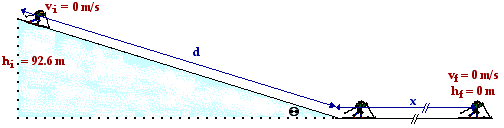The distance d along the incline is

d = hi / sin(theta) = 92.6 m / sin(19.2 deg) = 282 m

Now substitutions can be made into the work-energy equation and algebraic manipulation can be performed to solve for x:

PEi + Wnc = 0

PEi + Wincline + Wlevel = 0

(49.7 kg)•(9.8 m/s)•(92.6 m) + (40.2 N)•(282 m)•cos(180 deg) + (257 N)•(x)•cos(180 deg) = 0 J

45102 J - 11307 J - 257 x = 0 J

33795 J = 257 x

132 m = x

 Useful Web Links Analysis of Situations in Which Mechanical Energy is Conserved | Analysis of Situations Involving External Forces [ #1 | #2 | #3 | #4 | #5 | #6 | #7 | #8 | #9 | #10 | #11 | #12 | #13 | #14 | #15 | #16 | #17 | #18 | #19 | #20 | #21 | #22 | #23 | #24 | #25 | #26 | #27 | #28 | #29 | #30 | #31 | #32 | #33 | #34 | #35 | #36 | #37 | #38 | #39 | #40 | #41 | #42 | #43 | #44 | #45 ]

45. Mia Kneezhirt jumps from a second story dorm room (h = 7.91 m) to the ground below. Upon contact with the ground, she allows her 62.4-kg body to come to an abrupt stop as her center of gravity is displaced downwards a distance of 89.2 cm. Calculate the average upward force exerted by the ground upon Mia's fragile body. PSYW

 Answer: 5423 N This is another instance of a non-conservative force (the force of the ground upon Mia's body) doing work in order to alter the total mechanical energy. The relevant equation is KEi + PEi + Wnc = KEf + PEf Since Mia starts from rest, the KEi term is 0 J and can be canceled. If we designate the ground to be the zero level, then the PEf term is 0 J and can be canceled as well. Since Mia is finally stopped by the upward applied force of the ground, the KEf term is 0 J and can be canceled. The original equation then simplifies to PEi + Wnc = 0 J Expressions for potential energy and work can be substituted into this equation to change its form to m•g•hi + F•d•cos(theta) = 0 J All quantities are known except for F, so values can be substituted into the equation and algebra can be used to solve for F. The work is shown here. (62.4 kg) • (9.8 m/s/s) • (7.91 m) + F • (0.892 m) • cos(180 deg) = 0 4837 J - (0.892 m) • F = 0 4837 J = (0.892 m) • F F = 5423 N (Note that a critical assumption is made to make this problem solve-able. It is assumed that the Mia's center of mass starts a distance of 7.91 m above the position at which Mia's center of mass ultimately comes to rest. For most situations, this is usually a very safe assumption. In this case, the bending of Mia's knees would actually lower the center of gravity lower than the height that it would be at when she is just standing on the ground (in this case, 0.892 cm lower). Either this additional distance must be included in the PE term or it is assumed that Mia's landing involves somewhat of a spring action whereby she bends her knees to lower her body and then straightens up to an upright position - similar to the landing of an Olympic gymnast. The assumption is made in this problem that Mia utilizes the ground's force to not only stop her motion but to also elevate her back up to an upright position.)

 Useful Web Links Analysis of Situations in Which Mechanical Energy is Conserved | Analysis of Situations Involving External Forces [ #1 | #2 | #3 | #4 | #5 | #6 | #7 | #8 | #9 | #10 | #11 | #12 | #13 | #14 | #15 | #16 | #17 | #18 | #19 | #20 | #21 | #22 | #23 | #24 | #25 | #26 | #27 | #28 | #29 | #30 | #31 | #32 | #33 | #34 | #35 | #36 | #37 | #38 | #39 | #40 | #41 | #42 | #43 | #44 | #45 ]

#### Navigate to:

Review Session Home - Topic Listing

Work and Energy - Home || Printable Version || Questions with Links

Answers to Questions:  All || #1-10 || #11-25 || #26-36 || #37-45

## You Might Also Like ...

Users of The Review Session are often looking for learning resources that provide them with practice and review opportunities that include built-in feedback and instruction. If that is what you're looking for, then you might also like the following:

The Calculator Pad includes physics word problems organized by topic. Each problem is accompanied by a pop-up answer and an audio file that explains the details of how to approach and solve the problem. It's a perfect resource for those wishing to improve their problem-solving skills.

Visit: The Calculator Pad Home | Calculator Pad - Work and Energy

2. Minds On Physics the App Series

Minds On Physics the App ("MOP the App") is a series of interactive questioning modules for the student that is serious about improving their conceptual understanding of physics. Each module of the series covers a different topic and is further broken down into sub-topics. A "MOP experience" will provide a learner with challenging questions, feedback, and question-specific help in the context of a game-like environment. It is available for phones, tablets, Chromebooks, and Macintosh computers. It's a perfect resource for those wishing to refine their conceptual reasoning abilities. Part 3 of the series includes topics in Work and Energy.

Visit: MOP the App Home || MOP the App - Part 3